This is a follow up post to : Why SQL Fragmentation Remains High

As mentioned in this previous post, our dilemma was we were rebuilding every index on a nightly basis, and :

1. the process proves to be very resource intensive
2. we don’t see the benefit. The fragmentation for some of the indexes remain high.

After some digging, this is what we found:

Rebuild the index only if there will be at least 1000 pages affected. If < 1000 pages will be affected, fragmentation will not really be affected and potentially would remain high.

This is the script that we now use to selectively identify which indexes to rebuild, which indexes to reorganize, and which indexes to leave alone. This script uses the following criteria in determining which action to take:

• current fragmentation %
• number of pages used by the index

The current logic is:

• reorganize index : if fragmentation is > 10 % but < 30% and number of pages > 1000
• rebuild index : if fragmentation is > 30 and number of pages > 1000

This script is adapted from “Rebuild or reorganize indexes (with configuration)” from MSDN Books Online
(http://msdn.microsoft.com/en-us/library/ms188917.aspx)”

`   1: -- http://www.sqlmusings.com`
`   2: -- Ensure a USE <databasename> statement has been executed first.`
`   3: SET NOCOUNT ON`
`   4:`
`   5: -- adapted from "Rebuild or reorganize indexes (with configuration)" from MSDN Books Online `
`   6: -- (http://msdn.microsoft.com/en-us/library/ms188917.aspx)`
`   7:`
`   8: -- =======================================================`
`   9: -- || Configuration variables:`
`  10: -- || - 10 is an arbitrary decision point at which to`
`  11: -- || reorganize indexes.`
`  12: -- || - 30 is an arbitrary decision point at which to`
`  13: -- || switch from reorganizing, to rebuilding.`
`  14: -- || - 0 is the default fill factor. Set this to a`
`  15: -- || a value from 1 to 99, if needed.`
`  16: -- =======================================================`
`  17: DECLARE @reorg_frag_thresh   float        SET @reorg_frag_thresh   = 10.0`
`  18: DECLARE @rebuild_frag_thresh float        SET @rebuild_frag_thresh = 30.0`
`  19: DECLARE @fill_factor         tinyint    SET @fill_factor         = 80`
`  20: DECLARE @report_only         bit            SET @report_only         = 1`
`  21:`
`  22: -- added (DS) : page_count_thresh is used to check how many pages the current table uses`
`  23: DECLARE @page_count_thresh     smallint    SET @page_count_thresh   = 1000`
`  24:`
`  25: -- Variables required for processing.`
`  26: DECLARE @objectid       int`
`  27: DECLARE @indexid        int`
`  28: DECLARE @partitioncount bigint`
`  29: DECLARE @schemaname     nvarchar(130)`
`  30: DECLARE @objectname     nvarchar(130)`
`  31: DECLARE @indexname      nvarchar(130)`
`  32: DECLARE @partitionnum   bigint`
`  33: DECLARE @partitions     bigint`
`  34: DECLARE @frag           float`
`  35: DECLARE @page_count     int`
`  36: DECLARE @command        nvarchar(4000)`
`  37: DECLARE @intentions     nvarchar(4000)`
`  38: DECLARE @table_var      TABLE(`
`  39:                           objectid     int,`
`  40:                           indexid      int,`
`  41:                           partitionnum int,`
`  42:                           frag         float,`
`  43:                                   page_count   int`
`  44:                         )`
`  45:`
`  46: -- Conditionally select tables and indexes from the`
`  47: -- sys.dm_db_index_physical_stats function and`
`  48: -- convert object and index IDs to names.`
`  49: INSERT INTO`
`  50:     @table_var`
`  51: SELECT`
`  52:     [object_id]                    AS objectid,`
`  53:     [index_id]                     AS indexid,`
`  54:     [partition_number]             AS partitionnum,`
`  55:     [avg_fragmentation_in_percent] AS frag,`
`  56:     [page_count]                   AS page_count`
`  57: FROM`
`  58:     sys.dm_db_index_physical_stats (DB_ID(), NULL, NULL , NULL, 'LIMITED')`
`  59: WHERE`
`  60:     [avg_fragmentation_in_percent] > @reorg_frag_thresh`
`  61:     AND`
`  62:     page_count > @page_count_thresh`
`  63:     AND`
`  64:     index_id > 0`
`  65:`
`  66:`
`  67: -- Declare the cursor for the list of partitions to be processed.`
`  68: DECLARE partitions CURSOR FOR`
`  69:     SELECT * FROM @table_var`
`  70:`
`  71: -- Open the cursor.`
`  72: OPEN partitions`
`  73:`
`  74: -- Loop through the partitions.`
`  75: WHILE (1=1) BEGIN`
`  76:     FETCH NEXT`
`  77:         FROM partitions`
`  78:         INTO @objectid, @indexid, @partitionnum, @frag, @page_count`
`  79:`
`  80:     IF @@FETCH_STATUS < 0 BREAK`
`  81:`
`  82:     SELECT`
`  83:         @objectname = QUOTENAME(o.[name]),`
`  84:         @schemaname = QUOTENAME(s.[name])`
`  85:     FROM`
`  86:         sys.objects AS o WITH (NOLOCK)`
`  87:         JOIN sys.schemas as s WITH (NOLOCK)`
`  88:         ON s.[schema_id] = o.[schema_id]`
`  89:     WHERE`
`  90:         o.[object_id] = @objectid`
`  91:`
`  92:     SELECT`
`  93:         @indexname = QUOTENAME([name])`
`  94:     FROM`
`  95:         sys.indexes WITH (NOLOCK)`
`  96:     WHERE`
`  97:         [object_id] = @objectid AND`
`  98:         [index_id] = @indexid`
`  99:`
` 100:     SELECT`
` 101:         @partitioncount = count (*)`
` 102:     FROM`
` 103:         sys.partitions WITH (NOLOCK)`
` 104:     WHERE`
` 105:         [object_id] = @objectid AND`
` 106:         [index_id] = @indexid`
` 107:`
` 108:     -- Build the required statement dynamically based on options and index stats.`
` 109:     SET @intentions =`
` 110:         @schemaname + N'.' +`
` 111:         @objectname + N'.' +`
` 112:         @indexname + N':' + CHAR(13) + CHAR(10)`
` 113:     SET @intentions =`
` 114:         REPLACE(SPACE(LEN(@intentions)), ' ', '=') + CHAR(13) + CHAR(10) +`
` 115:         @intentions`
` 116:     SET @intentions = @intentions +`
` 117:         N' FRAGMENTATION: ' + CAST(@frag AS nvarchar) + N'%' + CHAR(13) + CHAR(10) +`
` 118:         N' PAGE COUNT: '    + CAST(@page_count AS nvarchar) + CHAR(13) + CHAR(10)`
` 119:`
` 120:     IF @frag < @rebuild_frag_thresh BEGIN`
` 121:         SET @intentions = @intentions +`
` 122:             N' OPERATION: REORGANIZE' + CHAR(13) + CHAR(10)`
` 123:         SET @command =`
` 124:             N'ALTER INDEX ' + @indexname +`
` 125:             N' ON ' + @schemaname + N'.' + @objectname +`
` 126:             N' REORGANIZE; ' +`
` 127:             N' UPDATE STATISTICS ' + @schemaname + N'.' + @objectname +`
` 128:             N' ' + @indexname + ';'`
` 129:`
` 130:     END`
` 131:     IF @frag >= @rebuild_frag_thresh BEGIN`
` 132:         SET @intentions = @intentions +`
` 133:             N' OPERATION: REBUILD' + CHAR(13) + CHAR(10)`
` 134:         SET @command =`
` 135:             N'ALTER INDEX ' + @indexname +`
` 136:             N' ON ' + @schemaname + N'.' +     @objectname +`
` 137:             N' REBUILD'`
` 138:     END`
` 139:     IF @partitioncount > 1 BEGIN`
` 140:         SET @intentions = @intentions +`
` 141:             N' PARTITION: ' + CAST(@partitionnum AS nvarchar(10)) + CHAR(13) + CHAR(10)`
` 142:         SET @command = @command +`
` 143:             N' PARTITION=' + CAST(@partitionnum AS nvarchar(10))`
` 144:     END`
` 145:     IF @frag >= @rebuild_frag_thresh AND @fill_factor > 0 AND @fill_factor < 100 BEGIN`
` 146:         SET @intentions = @intentions +`
` 147:             N' FILL FACTOR: ' + CAST(@fill_factor AS nvarchar) + CHAR(13) + CHAR(10)`
` 148:         SET @command = @command +`
` 149:             N' WITH (FILLFACTOR = ' + CAST(@fill_factor AS nvarchar) + ')'`
` 150:     END`
` 151:`
` 152:     -- Execute determined operation, or report intentions`
` 153:     IF @report_only = 0 BEGIN`
` 154:         SET @intentions = @intentions + N' EXECUTING: ' + @command`
` 155:         PRINT @intentions`
` 156:         EXEC (@command)`
` 157:     END ELSE BEGIN`
` 158:         PRINT @intentions`
` 159:     END`
` 160:     PRINT @command`
` 161:`
` 162: END`
` 163:`
` 164: -- Close and deallocate the cursor.`
` 165: CLOSE partitions`
` 166: DEALLOCATE partitions`
` 167:`
` 168: GO`
Share :
•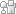•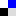•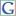••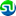•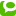•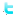Related posts: